### 二、常系数线性微分方程

1.  齐次线性微分方程通解的求法

[特征方程与特征根]  对于阶实常系数齐次线性微分方程

`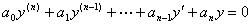(2)`

`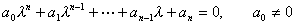(3)`

[齐次方程的通解]  为了求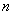阶常系数齐次线性微分方程(2)的通解，只要找出它的个线性无关的特解就可以了.根据其全体特征根的各种情况，分别列出对应的线性无关特解.

 特  征  根 对应的线性无关特解(j = 1,2,…,n)是互异实根 yj(x) =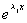(j = 1,2,…,n)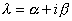是特征方程的单根，则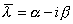也是特征方程的单根 y1(x) =cosβx y2(x) =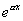sinβx是特征方程的r重实根 y1(x) =, y2(x) = x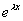,…， yr(x) = xr-1是特征方程的r重复根，则也是r重复根